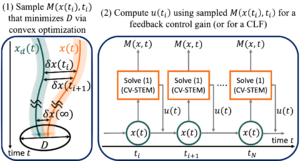# ConVex optimization-based Stochastic steady-state Tracking Error Minimization (CV-STEM)

We created a brand-new Website for this topic!Illustration of the CV-STEM control: M(x,t) denotes the optimal contraction metric; x(t) and xd(t) are controlled and desired system trajectories; u(t) is the control input computed by M(x,t).

CV-STEM is a new optimal state feedback control framework for a class of Itô stochastic nonlinear systems and Lagrangian systems.

CV-STEM Control
• Computes its control input by an optimal contraction metric M(x,t), which greedily minimizes an upper bound of the steady-state mean squared tracking error of the system trajectories (D of (1) in the figure above)
• Although the problem of minimizing D is originally nonlinear, its equivalent convex formulation is proposed utilizing state-dependent coefficient parameterizations of the nonlinear system equation (i.e. f(x,t) written as A(x,t)x)
•  Stochastic incremental contraction analysis shows that CV-STEM provides a sufficient guarantee for stability (exponential boundedness of the error for all time) with L2-robustness properties.
• The optimal contraction metric M(x,t) is exploited for state feedback control as depicted in (2) of the figure above

For the sake of its sampling-based implementation, we also present discrete-time stochastic contraction analysis with respect to a state- and time-dependent metric along with its explicit connection to continuous-time cases. The superiority of the CV-STEM to PID, H-infinity, and nonlinear control designed for spacecraft attitude control and synchronization problems is validated through numerical simulations. This work is available at https://arxiv.org/abs/2006.04359.